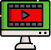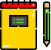VideosNotes

### Graphs Of Trigonometric Functions - 1

Graphs Of Trigonometric Functions
The six trigonometric functions are: Sine; Cosine; Tangent; Cosecant; Secant; Cotangent; Trigonometric graphs for these Trigonometry functions can be drawn if you know the following: Amplitude. It is the absolute value of any number multiplied with it on the trigonometric function.
Views: N/A
Video Duration: 10:19 (Part 1 of 2)